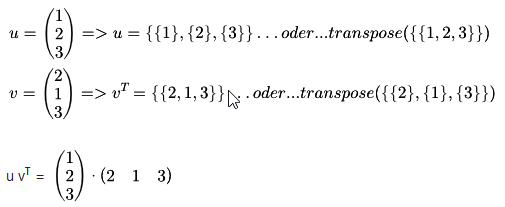# tensors and cross product

user3951 shared this question 3 years ago

In a cross product, like (1,0,0)⊗ (0,1,0) is used the symbol ⊗ of tensor product, that is not the same that ((0,1,0),(0,0,0),(0,0,0)).

A confusion is possible in advanced use or Geogebra.

Furio Petrossi1

Sorry, we won't be changing that1

For Tensor-Product you have to use matrices - perhaps it is possible to make the operator ⊗ work with matricesu ⊗ v^T1

Transpose command does'nt works for single column matrix (i.e. vector).

F.e. Transpose(Vector(1,3)) marks an error, so how can I do it?1

As I said, You have to convert vector to matrix. ggb vector is something different to list or matrix, but looks all alike

CAS only

{flatten(vector((1,3))}1

I understand. So in CAS there aren't problems.

In algebra it is possble to have access to single elements of an existing vector u only using x(y), y(u) as in points.

From vectors that have 2 or 3 elements it is possible create a matrix writing {{x(u), y(u)}} , but I'm not able to think up a simplest succession of commands, 'cause I cannot use the Element command with vectors.

Anyway, thank you very much

Furio1

I do not fully understand what your problem is. but you can also use Standard dot product

Sequence(Element(Identity(3),k)v,k,1,3)

If you do not know v = dim 2 or 3 you can use Dimension(v)

To access vector components and there are only vectors ( x,y) and (x,y,z) - nothing else?1

I used to consider vectors as a special case of matrix, but in geogebra it's different, because they must product a graphic array,Nothing else, thank you.

Furio